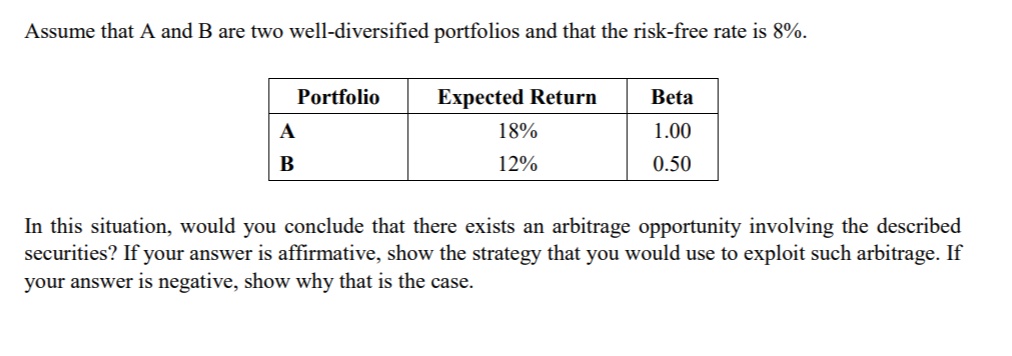Homework Help Question & Answers

# Please show all equations and work as needed. Assume that A and B are two well-diversified portfolios and that the risk-free rate is 8%. PortfolioExpected Returm 1.00 18% 12% 0.50 In this situation,...

Please show all equations and work as needed.Assume that A and B are two well-diversified portfolios and that the risk-free rate is 8%. PortfolioExpected Returm 1.00 18% 12% 0.50 In this situation, would you conclude that there exists an arbitrage opportunity involving the described securities? If your answer is affirmative, show the strategy that you would use to exploit such arbitrage. If your answer is negative, show why that is the case

According to A:

So, B's return should be=8%+0.5*10%=13%

But as B's return is 12%, so B is overvalued

Short sell B and buy/long A

##### Add Answer of: Please show all equations and work as needed. Assume that A and B are two well-diversified portfolios and that the risk-free rate is 8%. PortfolioExpected Returm 1.00 18% 12% 0.50 In this situation,...
More Homework Help Questions Additional questions in this topic.

• #### (TCO H) Audit risk consists of inherent risk, control risk, and detection risk. (a) Please completely define each of the above. (b) Indicate whether each of the statements below is true or false and e...

Need Online Homework Help?

Get FREE EXPERT Answers
WITHIN MINUTES
Related Questions